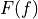# climpred.metrics._crps#

climpred.metrics._crps(forecast: xarray.Dataset, verif: xarray.Dataset, dim: Optional[Union[str, List[str]]] = None, **metric_kwargs: Any) [source]#

Continuous Ranked Probability Score (CRPS).

The CRPS can also be considered as the probabilistic Mean Absolute Error (mae). It compares the empirical distribution of an ensemble forecast to a scalar observation. Smaller scores indicate better skill.whereis the cumulative distribution function (CDF) of the forecast (since the verification data are not assigned a probability), and H() is the Heaviside step function where the value is 1 if the argument is positive (i.e., the forecast overestimates verification data) or zero (i.e., the forecast equals verification data) and is 0 otherwise (i.e., the forecast is less than verification data).

Note

The CRPS is expressed in the same unit as the observed variable. It generalizes the Mean Absolute Error (MAE), and reduces to the MAE if the forecast is determinstic.

Parameters
• forecast – Forecast with member dim.

• verif – Verification data without member dim.

• dim – Dimension to apply metric over. Expects at least member. Other dimensions are passed to xskillscore and averaged.

• metric_kwargs – optional, see xskillscore.crps_ensemble()

Notes

 minimum 0.0 maximum ∞ perfect 0.0 orientation negative

References

Example

>>> HindcastEnsemble.verify(
...     metric="crps", comparison="m2o", dim="member", alignment="same_inits"
... )
<xarray.Dataset>
Coordinates:
* init        (init) object 1954-01-01 00:00:00 ... 2005-01-01 00:00:00
* lead        (lead) int32 1 2 3 4 5 6 7 8 9 10
valid_time  (lead, init) object 1955-01-01 00:00:00 ... 2015-01-01 00:00:00
skill       <U11 'initialized'
Data variables:
SST         (lead, init) float64 0.1722 0.1202 0.01764 ... 0.05428 0.1638
Attributes: